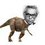# A nice result.

Given that $\quad a+b+c=0$

Prove that : $2(a^4+b^4+c^4)=(a^2+b^2+c^2)^2$

Nice proofs are always welcome!Note by Nihar Mahajan
6 years, 3 months ago

This discussion board is a place to discuss our Daily Challenges and the math and science related to those challenges. Explanations are more than just a solution — they should explain the steps and thinking strategies that you used to obtain the solution. Comments should further the discussion of math and science.

When posting on Brilliant:

• Use the emojis to react to an explanation, whether you're congratulating a job well done , or just really confused .
• Ask specific questions about the challenge or the steps in somebody's explanation. Well-posed questions can add a lot to the discussion, but posting "I don't understand!" doesn't help anyone.
• Try to contribute something new to the discussion, whether it is an extension, generalization or other idea related to the challenge.

MarkdownAppears as
*italics* or _italics_ italics
**bold** or __bold__ bold
- bulleted- list
• bulleted
• list
1. numbered2. list
1. numbered
2. list
Note: you must add a full line of space before and after lists for them to show up correctly
paragraph 1paragraph 2

paragraph 1

paragraph 2

[example link](https://brilliant.org)example link
> This is a quote
This is a quote
    # I indented these lines
# 4 spaces, and now they show
# up as a code block.

print "hello world"
# I indented these lines
# 4 spaces, and now they show
# up as a code block.

print "hello world"
MathAppears as
Remember to wrap math in $$ ... $$ or $ ... $ to ensure proper formatting.
2 \times 3 $2 \times 3$
2^{34} $2^{34}$
a_{i-1} $a_{i-1}$
\frac{2}{3} $\frac{2}{3}$
\sqrt{2} $\sqrt{2}$
\sum_{i=1}^3 $\sum_{i=1}^3$
\sin \theta $\sin \theta$
\boxed{123} $\boxed{123}$

Sort by:

Lets start off by finding the value of $\ a^2 +b^2 +c^2$ in terms of another sum by doing the following: $(a+b+c)^2 = a^2 +b^2 +c^2 +2(ab +ac +bc) = 0 \Rightarrow\ (a^2 +b^2 +c^2)^2 = 4(ab+ac+bc)^2$

Now expanding out the RHS: $... = 4(a^2 b^2 +a^2 c^2 + b^2 c^2 +2abc(a+b+c)) = 4(a^2 b^2 +a^2 c^2 + b^2 c^2)$

Now if we expand out $\ (a^2 +b^2 +c^2)^2$ and cancel out terms we get: $a^4 +b^4 +c^4 = 2 (a^2 b^2 +a^2 c^2 + b^2 c^2) \ ........ \ (1)$ $\Rightarrow\ 2(a^4 +b^4 +c^4) = a^4 +b^4 +c^4 + 2 (a^2 b^2 +a^2 c^2 + b^2 c^2)$

$\therefore\ 2(a^4 +b^4 +c^4) = (a^2 +b^2 +c^2)^2$

- 6 years, 3 months ago

Yeah! Thanks , I got an easier way . Read below.

- 6 years, 3 months ago

Approach: Factoring polynomials using the (slightly abused) remainder factor theorem for polynomials in several degrees.

Define $f(a, b, c) = 2 (a^4 + b^4 + c^4) - (a^2 + b^2 + c^2) ^2$.
We want to show that if $a+b+ c = 0$, then $f(a, b, c) = 0$. This strongly suggests to us that $f(a, b, c) = (a+b+c) \times g(a, b, c)$.

If $a + b + c = 0$, then we have $f( -b-c, b, c ) = 0$. Notice that the powers of $a$ only occur in even degree, hence $f(a, b, c) = f(-a, b, c)$. This implies that $f( b+c, b, c ) = 0$, and thus that $a-b-c \mid f(a, b, c)$. Cyclically, we also get that $b-c-a \mid f(a,b,c)$ and $c-a-b \mid f(a,b,c)$.

Putting this all together, this strongly suggests that

$(a+b+c)(a-b-c)(b-c-a)(c-a-b) \mid f(a,b,c)$

Since both sides have the same degree (4), we know that they differ by a constant. In fact, we can now verify that

$f(a, b, c) = - (a+b+c)(a-b-c)(b-c-a)(c-a-b)$

Staff - 6 years, 3 months ago

The equation $2(a^4 + b^4 + c^4) = (a^2 + b^2 + c^2)^2$ is equivalent to $-a^4 - b^4 - c^4 + 2a^2 b^2 + 2a^2 c^2 + 2b^2 c^2 = 0.$ This equation factors as $(a + b + c)(-a + b + c)(a - b + c)(a + b - c) = 0,$ and the result follows.

This factorization comes up in Heron's formula: \begin{aligned} K^2 &= s(s - a)(s - b)(s - c) \\ &= \frac{1}{16} (a + b + c)(-a + b + c)(a - b + c)(a + b - c) \\ &= \frac{1}{16} (-a^4 - b^4 - c^4 + 2a^2 b^2 + 2a^2 c^2 + 2b^2 c^2). \end{aligned}

- 6 years, 3 months ago

Great! That's the "Use Heron's Formula" that I alluded to. There are many ways to write the expression of $K$, and most people are only familiar with the factored form, instead of the expanded form.

Staff - 6 years, 3 months ago

Really great , I was curious of a heron's formula solution. Thanks!

- 6 years, 3 months ago

Use the convention of $S_1 = a+b+c$, $S_2=ab+bc+ca$ and $S_3 = abc$, and $P_n = a^n+b^n+c^n$. Then by Newton's Sum method:

$P_2 = S_1 P_1 - 2S_2 = 0 - 2S_2 = -2S_2$

$S_4 = S_1P_3-S_2P_2+S_3P_1 = 0 -S_2(-2S_2) + 0 = 2S_2^2$

Now $LHS = 2P_4 = 4S_2^2$ and $RHS = P_2^2 = (-2S_2)^2 = 4S_2^2 = LHS$

- 6 years, 3 months ago

Plug In 1+1-2 xD xD Just kidding. I'm on it!

- 6 years, 3 months ago

That's the most special case. -_-

- 6 years, 3 months ago